How Cheenta works to ensure student success?
Explore the Back-Story

# Sequences & Subsequences : IIT 2018 Problem 10# What are we learning?

Sequences, Subsequences are the key features in the field of real analysis. We will see how to imply these concepts in our problem

# Understand the problem

Let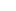= 1+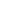++........+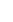for n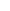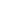Then which of the following is TRUE for the sequence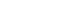:   (a)converges in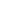.   (b)is a Cauchy sequence but does not converges to.   (c) The subsequence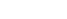is convergent in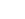when k is a even natural number.   (d)is not a Cauchy sequence. Difficulty Level Easy Suggested Book

I am going to give you 3 clues in the beginning you try to work out using them. Then I will elaborate this clues in the following hints  (I) Every convergent sequence is a Cauchy sequence  (II)Every subsequence of a convergent sequence is convergent  (III)Consider then term 1+++........+Does this remind you any well known series?

I wil start with (III) consider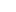=1+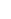+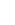+........+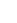Isn't the seris that we have to , is the value at x=1. Hence the given series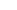e\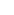So option (a) is incorrect.

Every subsequence of a convergent sequence is convergent sois convergent not only for even k, but for any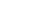. So option (c) is incorrect.

Every convergent sequence is a Cauchy sequence so option (d) is incorrect and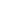so the given subsequence is convergent in. So only option (b) is correct.

# Connected Program at Cheenta

The higher mathematics program caters to advanced college and university students. It is useful for I.S.I. M.Math Entrance, GRE Math Subject Test, TIFR Ph.D. Entrance, I.I.T. JAM. The program is problem driven. We work with candidates who have a deep love for mathematics. This program is also useful for adults continuing who wish to rediscover the world of mathematics.

# What are we learning?

Sequences, Subsequences are the key features in the field of real analysis. We will see how to imply these concepts in our problem

# Understand the problem

Let= 1+++........+for nThen which of the following is TRUE for the sequence:   (a)converges in.   (b)is a Cauchy sequence but does not converges to.   (c) The subsequenceis convergent inwhen k is a even natural number.   (d)is not a Cauchy sequence. Difficulty Level Easy Suggested Book

I am going to give you 3 clues in the beginning you try to work out using them. Then I will elaborate this clues in the following hints  (I) Every convergent sequence is a Cauchy sequence  (II)Every subsequence of a convergent sequence is convergent  (III)Consider then term 1+++........+Does this remind you any well known series?

I wil start with (III) consider=1+++........+Isn't the seris that we have to , is the value at x=1. Hence the given seriese\So option (a) is incorrect.

Every subsequence of a convergent sequence is convergent sois convergent not only for even k, but for any. So option (c) is incorrect.

Every convergent sequence is a Cauchy sequence so option (d) is incorrect andso the given subsequence is convergent in. So only option (b) is correct.

# Connected Program at Cheenta

The higher mathematics program caters to advanced college and university students. It is useful for I.S.I. M.Math Entrance, GRE Math Subject Test, TIFR Ph.D. Entrance, I.I.T. JAM. The program is problem driven. We work with candidates who have a deep love for mathematics. This program is also useful for adults continuing who wish to rediscover the world of mathematics.

This site uses Akismet to reduce spam. Learn how your comment data is processed.

### Knowledge Partner